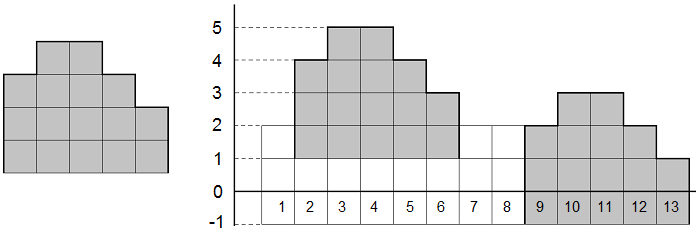# CF471D MUH and Cube Walls 题解【KMP】【差分】【构造】

一不小心看到了一句话题解就做出来了。感觉很浪费，把思路记录一下以后看。。。

## Description

Polar bears Menshykov and Uslada from the zoo of St. Petersburg and elephant Horace from the zoo of Kiev got hold of lots of wooden cubes somewhere. They started making cube towers by placing the cubes one on top of the other. They defined multiple towers standing in a line as a wall. A wall can consist of towers of different heights.

Horace was the first to finish making his wall. He called his wall an elephant. The wall consists of w towers. The bears also finished making their wall but they didn’t give it a name. Their wall consists of n towers. Horace looked at the bears’ tower and wondered: in how many parts of the wall can he “see an elephant”? He can “see an elephant” on a segment of w contiguous towers if the heights of the towers on the segment match as a sequence the heights of the towers in Horace’s wall. In order to see as many elephants as possible, Horace can raise and lower his wall. He even can lower the wall below the ground level (see the pictures to the samples for clarification).

Your task is to count the number of segments where Horace can “see an elephant”.

## Input

The first line contains two integers n and w (1 ≤ n, w ≤ 2·105) — the number of towers in the bears’ and the elephant’s walls correspondingly. The second line contains n integers ai (1 ≤ ai ≤ 109) — the heights of the towers in the bears’ wall. The third line contains w integers bi (1 ≤ bi ≤ 109) — the heights of the towers in the elephant’s wall.

## Output

Print the number of segments in the bears’ wall where Horace can “see an elephant”.

## Examples

input
13 5
2 4 5 5 4 3 2 2 2 3 3 2 1
3 4 4 3 2
output
2

## Note

The picture to the left shows Horace’s wall from the sample, the picture to the right shows the bears’ wall. The segments where Horace can “see an elephant” are in gray.## 题意：

给出一段轮廓和一段墙壁，问墙壁上是否存在一段这样的轮廓。

## 题解：

一种暴力的思想是枚举初始高度，把所有高度都减去这个值，然后匹配另一段。用KMP可以做到$O(\max\limits_{i\in [1,n]} {a_i}(n+m))$，但是显然不在我们的可行范围内。

考虑到匹配的段是连续的，就可以用差分来做。但是第一个数怎么办呢？实际上我们把长为$m$的段转为长为$(m-1)$的差分段，不管第一段和前面一段，然后用差分匹配。不过有细节需要注意，就是A串的第一个同样要被合并到后面，或者把a定义为$-\infty$，就可以避免一些极端情况如1,2,3,4,5，而a默认=0，就会多统计一次（等差数列也都会这样）。（前面一种处理方法稳一些）

## Code：

#include<cstdio>
#include<cstring>
int n,m;
int s,t;
int nxt;
int main()
{
scanf("%d%d",&n,&m);
for(int i=1;i<=n;++i)
scanf("%d",&s[i]);
s=-10000000;//避免特殊情况
for(int i=n;i;--i)//计算差分
s[i]=s[i]-s[i-1];
for(int i=1;i<=m;++i)
scanf("%d",&t[i]);
for(int i=m;i;--i)
t[i]=t[i]-t[i-1];
for(int i=1;i<m;++i)
t[i]=t[i+1];
--m;//注意B数组缩小了
for(int i=2,j=0;i<=m;++i)//nxt数组计算
{
while(j&&t[j+1]!=t[i])
j=nxt[j];
if(t[j+1]==t[i])
++j;
nxt[i]=j;
}
int cnt=0;
for(int i=1,j=0;i<=n;++i)//KMP过程
{
while(j&&(j==m||t[j+1]!=s[i]))
j=nxt[j];
if(t[j+1]==s[i])
++j;
if(j==m)
++cnt;
}
printf("%d\n",cnt);
return 0;
}


### 说点什么Subscribe

/* */